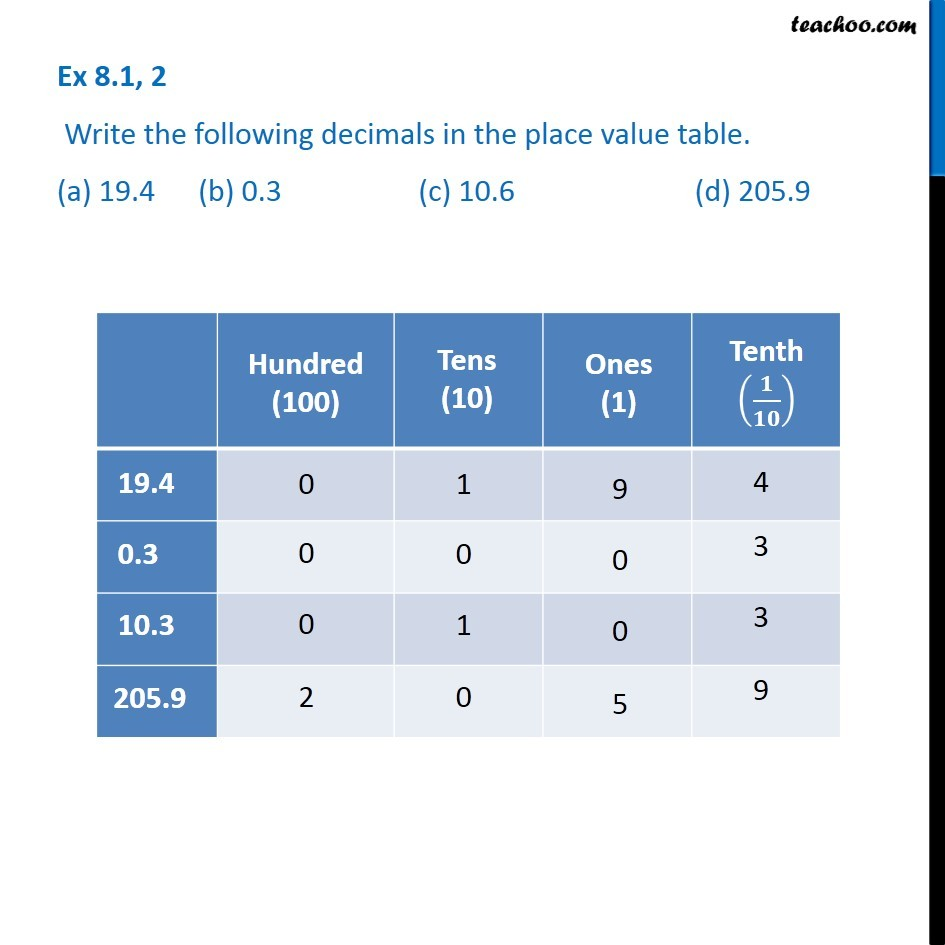Subscribe to our Youtube Channel - https://you.tube/teachoo

1. Chapter 8 Class 6 Decimals
2. Concept wise
3. Tenth

Transcript

Ex 8.1, 2 - Chapter 8 Class 6 Decimals Write the following decimals in the place value table. (a) 19.4 (b) 0.3 (c) 10.6 (d) 205.9 For (a) Hundred (100) = 0 Tens (10) = 1 Ones (1) = 9 Tenths (1/10) = 4 For (b) Hundred (100) = 0 Tens (10) = 0 Ones (1) = 0 Tenths (1/10) = 3 For (c) Hundred (100) = 0 Tens (10) = 1 Ones (1) = 0 Tenths (1/10) = 3 For (d) Hundred (100) = 2 Tens (10) = 0 Ones (1) = 5 Tenths (1/10) = 9

Tenth